# Writing Standard-Form Equations for Parabolas: Definition & Explanation

An error occurred trying to load this video.

Try refreshing the page, or contact customer support.

Coming up next: Rational Exponents

### You're on a roll. Keep up the good work!

Replay
Your next lesson will play in 10 seconds
• 0:05 Standard Form Equation…
• 0:53 Vertext Form Equation…
• 1:28 Finding the Equation…
• 3:29 Converting Vertex Form…
• 5:44 Lesson Summary

Want to watch this again later?

Timeline
Autoplay
Autoplay
Speed

#### Recommended Lessons and Courses for You

Lesson Transcript
Instructor: Yuanxin (Amy) Yang Alcocer

Amy has a master's degree in secondary education and has taught math at a public charter high school.

The standard form equation for parabolas is one of the two ways to write parabola equations. Learn what the other one is and how it comes into play when writing standard form equations for parabolas.

## Standard Form Equation for Parabola

The standard form equation for parabolas looks like your standard quadratic: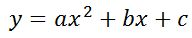This form provides you a couple of key bits of information.

1. The first number you see, a, tells you whether your parabola opens up or down. If a is negative, it will open downwards and look like a frown. If 'it is positive, it will open up and will look like a smile. A good way to remember this is to think of the phrase, 'Be positive; don't frown.'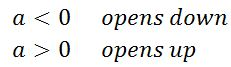2. Using both a and b will give you the axis of symmetry of the parabola. The axis of symmetry of the parabola is the line that acts as a mirror for the parabola. The parabola on either side of the axis of symmetry is the mirror image of the other side. The formula for finding the axis of symmetry from the standard form equation is: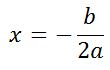## Vertex Form Equation for Parabola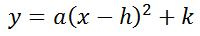The other way of writing the equation for a parabola is the vertex form. The vertex form gives you three bits of information about the parabola.

1. Just like the standard form, the first number, a, tells you whether the parabola opens up or down. If it's positive, it opens up and if it's negative, it opens down.
2. The number h gives you the axis of symmetry, x = h.
3. The vertex form also gives you the vertex or tip of the parabola, (h, k).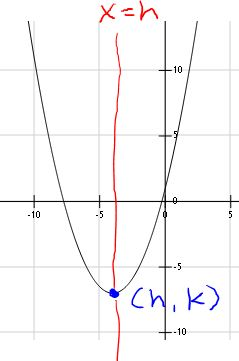## Finding the Equation in Vertex Form

Now that we've covered our definitions, let's see how this works. We are given a parabola with a vertex of (1, 2) and another point (0, 5), and we're asked to find the standard form equation. What do we do?

We can't figure out the standard form directly using this information. This is where the vertex form comes in. In order to write the standard form equation, we first need to write the equation in vertex form because the information provided allows us to completely figure out the parabola's equation using that form.

The coordinates of the vertex are (1, 2), which correspond to the values (h, k). So we know that in this case, h = 1 and k = 2.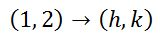We can plug those values into our vertex form equation for h and k.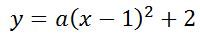The only value we still need to find is a, but how do we do that? We were given the coordinates of the vertex, which we already used, and the coordinates of another point on the parabola (0, 5). We know that those coordinates are the x- and y-values of the parabola at that point, so let's plug in x = 0 and y = 5, and then solve the entire equation for a.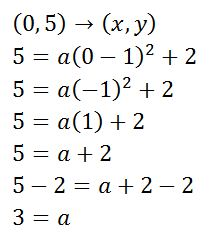Once we've plugged in our x- and y-values, we can simplify what's in the parentheses, take the square, and subtract the 2 from both sides to end up with a value of 3 for a. We now have our quadratic equation in vertex form.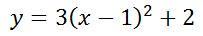The next step is to make the conversion from vertex form to standard form.

## Converting Vertex Form into Standard Form

Remember that our standard form equation for a parabola will be a standard quadratic:To unlock this lesson you must be a Study.com Member.

### Register to view this lesson

Are you a student or a teacher?

### Unlock Your Education

#### See for yourself why 30 million people use Study.com

##### Become a Study.com member and start learning now.
Back
What teachers are saying about Study.com

### Earning College Credit

Did you know… We have over 160 college courses that prepare you to earn credit by exam that is accepted by over 1,500 colleges and universities. You can test out of the first two years of college and save thousands off your degree. Anyone can earn credit-by-exam regardless of age or education level.